# Knowledge Base

## Solution 30130: When Using Symbolic Pi, Mixed Number Conversions are Improperly Displayed on the TI-36X Pro and TI-30X Pro MultiView™ Calculators.

### When using symbolic Pi, why are improper fractions and mixed numbers not converting properly on my TI-36X Pro or TI-30X Pro MultiView calculator?

Issue:

When you do a calculation using symbolic pi, you will get an approximate decimal answer to the number of decimal places carried by a calculator. If you convert the decimal result (stored in the calculator) to a mixed number and then repeatedly toggle back and forth between the decimal and mixed number result, the display of the mixed number, and thus the interpretation of that result, is not correct. Once you do this, any other mixed fractions that include pi calculations you attempt to do will inaccurately display.

Sample problem: Area of a Circle: Not all screens are shown.

• Enter a calculation with Pi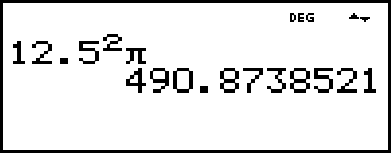• Toggle to fraction result. The Improper fraction result is correctly displayed.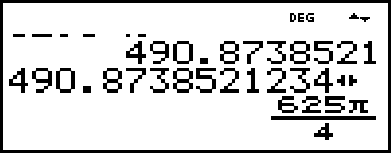• Use >n/d<>U n/d (fraction to mixed fraction).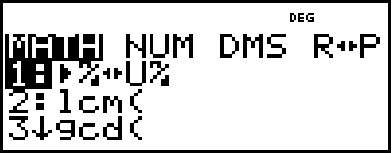• The fraction to mixed fraction toggle does not convert (Expected)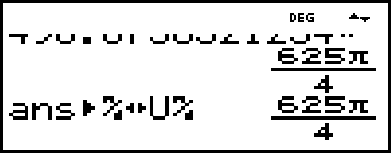• Toggle fraction approximation back to decimal approximation. You get the expected results.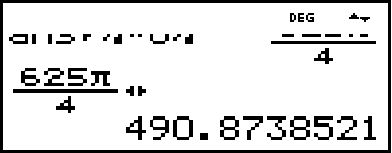• After several presses of toggle you may see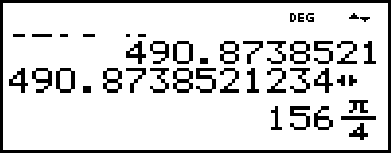The correct display should be 156 ¼ p. The p in the display is in the wrong location.

From this state, it is best to reset the calculator. (Please see Workaround below.) Always look to see if an answer makes sense. In this case, the fraction to mixed fraction conversion incorrectly tried to do the conversion and the display is incorrect because p was pulled into the numerator of the mixed number.

Note: If you directly enter the mixed number 156 p/4 as a mixed number using the mixed number template over the 7 key, pressing enter will give you an error because this is not a valid mixed number format on the TI-36X Pro or TI-30X Pro MultiView calculator!

Workaround:

Do a hard reset of the calculator to resolve the problem. To reset the unit, hold down the [On] key and then press the [Clear] key. This action will clear and reset the memory. You will be able to do calculations again with accuracy. [2nd] [reset] 2 or pressing the reset button on the back of the calculator will also perform a hard reset (factory defaults).